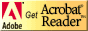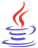Standardized Test Preparation and Tips for Success

Strand Resources: Patterns, Functions, and AlgebraCT4ME has identified resources related to each benchmark of the Ohio Mathematics Academic Content Standards that students should have mastered by the end of the grades 8-10 program.

Identify the benchmark (A-J) for review in Patterns, Functions, and Algebra.  Below the benchmark, you will find Web resources for reviewing the concept and practice problems.

Students should be able to review on their own using these resources.  If you are not an Ohio educator, these resources can still benefit your test prep efforts, as benchmarks in other states are similarly stated.

Patterns, Functions and Algebra (pdf) This test prep booklet (about 200 KB) is designed to help you monitor your progress toward mastery of each benchmark in this strand.  You can print the entire booklet, or just those pages for benchmarks you need to work on.  Write notes in the booklet next to each resource you use in your review.If needed, download Adobe Acrobat Reader, free software for viewing and printing PDF files.You might need to download Java, a free plug-in to interact with some virtual manipulatives on this page.Other strand resources:

Number, Number Sense, and Operations ]   [ Measurement ]   [ Geometry and Spatial Sense ]   [ Patterns, Functions, and Algebra ]   [ Data Analysis and Probability ]   [ Mathematical Processes ]What is Algebra?Benchmarks in Patterns, Functions, and AlgebraBenchmarks in this strand have been correlated to the Common Core State Standards (CCSS) for Mathematics for the Conceptual Category (CC), Domain (D), and Cluster (C).

A.  Generalize and explain patterns and sequences in order to find the next term and the nth term.

CCSS: CC: Functions, D: Interpreting Functions, C: Understand the concept of a function and use function notation.

CCSS: CC: Functions, D: Build Functions, C: Build a function that models a relationship between two quantities.

Purplemath: Number Patterns finding the next number in a sequence, including common differences and recursions.

Mathguide.com:Play video at YouTube.com:

B.  Identify and classify functions as linear or nonlinear, and contrast their properties using tables, graphs or equations.

CCSS: CC: Functions, D: Interpreting Functions, C: Analyze functions using different representations.

CCSS: grade 8, D: Functions, C: Define, evaluate, and compare functions.

CCSS: grade 8, D: Functions, C: Use functions to model relationships between quantities.

Purplemath: FunctionsPlay video at YouTube.com:

C.  Translate information from one representation (words, table, graph or equation) to another representation of a relation or function.

CCSS: CC: Algebra, D: Reasoning with Equations and Inequalities, C: Represent and solve equations and inequalities graphically.

CCSS: CC: Functions, D: Interpreting Functions, C: Interpret functions that arise in applications in terms of the context, and C: Analyze functions using different representations.

CCSS: grade 8, D: Functions, C: Define, evaluate, and compare functions.

D.  Use algebraic representations, such as tables, graphs, expressions, functions and inequalities, to model and solve problem situations.

CCSS: CC: Algebra, D: Creating Equations, C: Create equations that describe numbers or relationship.

CCSS: CC: Functions, D: Linear, Quadratic, and Exponential Models, C: Construct and compare linear, quadratic, and exponential models and solve problems.

CCSS: grade 8, D: Functions, C: Use functions to model relationships between quantities.

Mathguide.com: The quizmaster for each of the following is fill in and check answers:

OnlineMathLearning.com: Types of Algebra Word Problems Among those are complete explanations and how to's for age, average, coin, consecutive integer, distance, fraction, geometry, interest, lever, mixture, number sequences, ratio, proportion, symbol, and work problems.

Purplemath: Translating Word Problems ApplicationsPlay the YouTube videos from the Ohio Resource Center Tutorials for High School Mathematics:Play videos from Khan Academy:Play videos at YouTube.com

• Distance = Rate x Time (Airplanes traveling in opposite directions)

• Coins (coins consisting of dimes and nickels)

• Rate of Work Problems (How much time does it take to complete the job if two people or things work together?)

• Investments (Use Interest =Principal x Rate: Money was invested, part at one rate and part at another rate.  The total interest in one year is provided.  How much was invested at each rate?)

E.  Analyze and compare functions and their graphs using attributes, such as rates of change, intercepts and zeros.

CCSS: CC: Functions, D: Interpreting Functions, C: Interpret functions that arise in applications in terms of the context, and C: Analyze functions using different representations.

CCSS: CC: Functions, D: Build Functions, C: Build new functions from existing functions.

Purplemath: Graphing Overview

Shodor Interactivate, Virtual Manipulative: Function Flyer -- plot an algebraic function on the coordinate plane, and use sliders to manipulate the constants of the graphed function.

F.  Solve and graph linear equations and inequalities.

CCSS: CC: Algebra, D: Reasoning with Equations and Inequalities, C: Understand solving equations as a process of reasoning and explain the reasoning, C: Solve equations and inequalities in one variable, and C: Represent and solve equations and inequalities graphically.

CCSS: CC: Functions, D: Interpreting Functions, C: Analyze functions using different representations.

CCSS: CC: grade 8, D: Expressions and Equations, C: Analyze and solve linear equations and pairs of simultaneous linear equations.

Mathguide.com: Solve linear equations of the form ax + b =c; ax + b + cx + d; and ax + b = cx +d.  The page contains explanations and quizmaster for each type.  Fill in and check answers.

Purplemath:

The Math Page: Algebra, Section 32, Equation of a straight line, including graphing using intercepts, y = ax, and lines parallel to axes, explanations, plus problems with answers

Shodor Interactivate:

• Algebra Quiz: Practice solving algebraic equations.  The quiz allows you to select the difficulty level, time limit and equation type. Choose to include one or more options: variable on both sides, distributive property, quadratic, one-step problems, and two-step problems.
• Graphit: Graph linear equations and inequalities.Play videos at YouTube.com:

G.  Solve quadratic equations with real roots by graphing, formula and factoring.

CCSS: CC: Algebra, D: Seeing Structure in Expressions, C: Write expressions in equivalent forms to solve problems.

CCSS: CC: Algebra, D: Reasoning with Equations and Inequalities, C: Solve equations and inequalities in one variable.

CCSS: CC: Geometry, D: Expressing Geometric Properties with Equations, C: Translate between the geometric description and the equation of a conic section.

Mathguide.com:

The Math Page: Algebra, Section 36, Solve Quadratic Equations by graphing, formula, factoring, explanations, plus problems with answersPlay videos at YouTube.com:

H.  Solve systems of linear equations involving two variables graphically and symbolically.

CCSS: CC: Algebra, D: Reasoning with Equations and Inequalities, C: Solve systems of equations, and C: Represent and solve equations and inequalities graphically.

CCSS: CC: grade 8, D: Expressions and Equations, C: Analyze and solve linear equations and pairs of simultaneous linear equations.

Mathguide.com: Solve systems of linear equations with addition, multiplication/addition, and substitution.  Lesson and then quizmaster.  Fill in and check answers.

Purplemath: Systems of Linear Equations

The Math Page: Algebra, Section 33, Simultaneous Linear Equations,  explanations for graphical, addition, substitution methods, plus problems with answersPlay videos at YouTube.com:

I.  Model and solve problem situations involving direct and inverse variation.

Purplemath: Direct, Joint, and Inverse VariationPlay videos at YouTube.com for solving algebra word problems.  The presenter translates the word problem into the algebraic equation, discusses the problem, shows diagrams as needed, and presents the solution.  He is very detailed--excellent how-to videos.  Students: For a learning experience, pause the video after the problem is presented, then solve.  Use the video to check your solution method:

J.  Describe and interpret rates of change from graphical and numerical data. (Includes: Compute and interpret slope, midpoint, and distance given a set of ordered pairs.)

CCSS: CC: Functions, D: Interpreting Functions, B: Interpret functions that arise in applications in terms of the context.

Purplemath: Meaning of Slope and Y-Intercept

AlgebraLab.org: Algebra I: Distance and Midpoint Formulas.  Study aids with examples and practice problems (short answer and T/F).  Given two ordered pairs, determine length of a segment and midpoint.  Given three ordered pairs, determine if the vertices form a right triangle.Are you ready for the test?

References

*Ohio Mathematics Academic Content Standards (2001). http://www.ode.state.oh.us/GD/Templates/Pages/ODE/ODEPrimary.aspx?page=2&TopicID=1696&TopicRelationID=1704 [URL is no longer active.]

Ohio Mathematics Academic Content Standards Crosswalk, K-8 and High School (2010).  https://web.archive.org/web/20180206120934/http://education.ohio.gov/getattachment/Topics/Ohio-s-New-Learning-Standards/Mathematics/Transition-Tools-Ohio-s-New-Learning-Standards-K-1/Alignment-Toolkit-Phase-1-Gap-Analysis/HS-crosswalk-mathematics-4-17-12.pdf.aspx [Revised 4/17/2012].

*Note 2019: The current academic content standards for mathematics are now available at http://education.ohio.gov/Topics/Learning-in-Ohio/Mathematics/Ohio-s-Learning-Standards-in-MathematicsSee other strand resources:See related pages: [ Standardized Test Preparation and Tips for Success (first  page)]  [ OGT Introduction ]